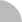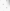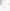# 臺灣博碩士論文加值系統

(44.200.82.149) 您好！臺灣時間：2023/06/03 23:22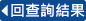:::

### 詳目顯示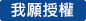: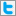Twitter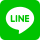•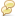被引用:0
•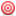點閱:138
•評分: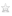•下載:0
•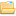書目收藏:0
 邏輯是數學裡專門探討敘述的推理演繹的一個分支，被認定為推理的研究。由於數學的本質是由關於數學物件的敘述以及驗證這些敘述的證明所構成，因此，整個數學領域可以用邏輯加以分析。而理論電腦科學的核心部分---演算法，其觀念的基礎就是計算的觀念，也是個數學物件，可由邏輯分析之。在這篇論文裡，我們提供了邏輯的基本性質，並且利用這些性質來研究一些計算問題，特別是漢彌爾頓路徑(Hamiltonian Path)這個圖型理論的問題。
 Logic is a branch of mathematics that investigates the deductions about statements and is recognizedas the study of reasoning. Because of this, the whole mathematics can be investigatedby logic and is even governed by it since the essentials of mathematics consist of statementsabout mathematical objects and the proofs that verify these statements. Since the underlyingconcept of algorithms, the critical part of theoretical computer science, is that of computation,which is also a mathematical object, it can aslo be analyzed by logic. In this thesis we providethe basic properties of logic, and then use them to investigate some computational problems,especially the graph-theoretic problem Hamiltonian path.
 1 Introduction 11.1 Prolog . . . . . . . . . . . . . . . . . . . . . . . . . . . . . . . . . . . . . . . . . 11.2 Categories inside Logic . . . . . . . . . . . . . . . . . . . . . . . . . . . . . . . . 21.2.1 Computational Problems . . . . . . . . . . . . . . . . . . . . . . . . . . . 22 Topics on Propositional Logic 42.1 Preliminaries . . . . . . . . . . . . . . . . . . . . . . . . . . . . . . . . . . . . . 52.2 Syntax . . . . . . . . . . . . . . . . . . . . . . . . . . . . . . . . . . . . . . . . . 62.3 Semantics . . . . . . . . . . . . . . . . . . . . . . . . . . . . . . . . . . . . . . . 92.3.1 Equvalence between Propositions . . . . . . . . . . . . . . . . . . . . . . 122.4 Deduction Systems . . . . . . . . . . . . . . . . . . . . . . . . . . . . . . . . . . 153 Topics on Predicate Logic 193.1 First-order Logic . . . . . . . . . . . . . . . . . . . . . . . . . . . . . . . . . . . 203.1.1 Syntax . . . . . . . . . . . . . . . . . . . . . . . . . . . . . . . . . . . . . 203.1.2 Semantics . . . . . . . . . . . . . . . . . . . . . . . . . . . . . . . . . . . 233.1.3 Deduction Systems . . . . . . . . . . . . . . . . . . . . . . . . . . . . . . 263.1.4 Weakness of First-Order Logic . . . . . . . . . . . . . . . . . . . . . . . . 293.2 Second-Order Logic . . . . . . . . . . . . . . . . . . . . . . . . . . . . . . . . . . 293.3 Remarks . . . . . . . . . . . . . . . . . . . . . . . . . . . . . . . . . . . . . . . . 304 Graph-Theoretic Problems as Expressed in Logical Formulae 314.1 Prolog . . . . . . . . . . . . . . . . . . . . . . . . . . . . . . . . . . . . . . . . . 314.2 The Successor Function in Graph-Theoretic Problems . . . . . . . . . . . . . . . 325 Concluding Remarks 37Bibliography 39
  R. Fagin Generalized rst-order spectra and polynomial-time recognizable sets," pp. 43{73 in Complexity of Computation, edited by R. M. Karp SIAM-AMS Proceedings, vol. 7,1974. P. Kolatis and M. Vardi { 1 laws and decision problems for fragments of second-orderlogic," Proc. 3rd IEEE Symp. on Logic In Comp. Sci, pp. 2{11, 1988. N. Immerman Relational queries computable in polynomial time," Information and Control,68, pp. 86{104, 1986. M. Y. Vardi The complexity of relational query languages," Proceedings of the 14th ACMSymp. on the Theory of Computing, pp. 137{146, 1982. C. H. Papadimitriou A note on the expressive power of PROLOG," Bull. of the EATCS,26, pp. 21{23, 1985. C. H. Papadimitriou Computational complexity, second edition, Addison-Wesley Longman. E. Gradel The expressive power of second-order Horn logic," Proc. 8th Symp. on Theor.Aspects of Comp. Sci., vol. 480 of Lecture Notes in Computer Science, pp. 466{477, 1991. H.-D. Ebbinghaus, J. Flum, and W. Thomas Mathematical logic, second edition, Springer. H. B. Enderton A Mathematical Introduction to Logic, Academic Press. D. van Dalen Logic and Structure, fourth edition, Springer. R. P. Grimaldi Discrete and Combinatorial Mathematics, an Applied Introduction, fthedition, Pearson Addison-Wesley. E. Mendelson Introduction to Mathematical Logic, fourth edition, Chapman & Hall/CRC. L. Henkin The completeness of rst-order functional calculus," J. Symb. Logic, 14, pp.159 { 166, 1949. K. Godel Die Vollstandigkeit der Axiome der Logischen Funktionenkalkuls" (The completenessof the axioms of the logical function calculus), Monat, Math. Physik, 37, pp. 349{ 360, 1930. K. Godel Uber formal unentscheidbare Satze der Principia Mathematica und verwandterSysteme" (On formally undecidable theorems in Principia Mathematica and related systems),Monatschefte fur Mathematik und Physik, 38, pp. 173 { 198, 1931 L. Henkin, J. D. Monk and A. Tarski Cylindric Algebras, North Holland, 1971 { 1985. T. H. Cormen, C. E. Leiserson, R. L. Rivest and C. Stein Introduction to Algorithms,second edition, MIT Press, Cambridge, Massachusetts, 2001 E. Horowitz, S. Sahni, D. Mehta Fundamentals of Data Structures in C++, ComputerScience Press, An imprint of W. H. Freeman and Company, New York, 1995. S. Hedman A rst course in logic : an introduction to model theory, proof theory, computability,and complexity, Oxford, New York, Oxford University Press, 2004. S. Russell, P. Norvig Arti cial Intelligence, a Modern Approach, second edition, PrenticeHall Series in Arti cial Intelligence, 2003.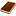國圖紙本論文推文當script無法執行時可按︰推文 網路書籤當script無法執行時可按︰網路書籤 推薦當script無法執行時可按︰推薦 評分當script無法執行時可按︰評分 引用網址當script無法執行時可按︰引用網址 轉寄當script無法執行時可按︰轉寄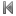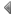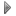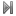top
 無相關論文

 無相關期刊

 1 任務導向平衡訓練合併感覺操弄對腦中風患者平衡功能與姿勢控制之療效研究 2 自動化網路安全防禦系統 3 應用壓阻式微懸臂梁生物感測器偵測巴斯德桿菌之研究 4 虛擬化多核心之省電投射技術 5 以統計方法來自動偵測線上遊戲機器人程式 6 經驗為良師?股市崩盤期之投資人行為分析 7 側掃聲納回散射訊號之海床地貌影像分析研究 8 中雷諾數流場移動物體之數值計算 9 應用實質選擇權決定埤塘擴充時程之研究 10 流感病毒株同期競爭現象與當代流感疫苗政策之數理模建 11 實現熱門配對的演算法設計及複雜度分析 12 居家制動療法於腦性麻痺兒童動作功能、日常生活功能、親職壓力及生活品質之療效研究 13 實質選擇權應用於股價與盈餘關係之研究 14 本益比、市淨率與投資報酬關係之研究 15 美沙冬維持療法患者之酒精使用問題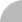簡易查詢 | 進階查詢 | 熱門排行 | 我的研究室